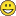# QlikView App Development

Discussion Board for collaboration related to QlikView App Development.

Announcements
If you are a Qlik Insight Bot user, join this collaborative group: JOIN GROUP
Highlighted
New Contributor

## Cell value in straight table

I have a raw data like below table and need below data, could you please show me how to do it. Many thanks

First,  top2 AMT from MAX(Date) => 500000 and 70000

Second,  "Device Name" of top2 AMT => TT and BB

Third,  difference AMT between MAX(Date) and MAX(Date)-1

It should be :

500000 ,   TT      , 493990 (500000-6010)

70000   ,    BB    , 70000 (70000-0)1 Solution

Accepted Solutions
HighlightedMVP

## Re: Cell value in straight table

Like this:The expression for AMT is:

Sum( {<R#={"=Rank( Sum({<Date={'\$(=Date(Max(Date)))'}>} AMT))<=2"}>} AMT )

NOTE: the R# is a field which is created in the load script in this way:

RowNo() AS R#,

"Device Name",

.....

......;

The expression for AMT1-AMT2:

Column(1)- Sum( {1<Date={'\$(=Date(Max(Date)-1))'},[Device Name]=P({<R#={"=Rank( Sum({<Date={'\$(=Date(Max(Date)))'}>} AMT))<=2"}>})>} AMT )

( Column(1) could be replace by the full expression for AMT or even replaced with just AMT )

2 Replies
HighlightedMVP

## Re: Cell value in straight table

Like this:The expression for AMT is:

Sum( {<R#={"=Rank( Sum({<Date={'\$(=Date(Max(Date)))'}>} AMT))<=2"}>} AMT )

NOTE: the R# is a field which is created in the load script in this way:

RowNo() AS R#,

"Device Name",

.....

......;

The expression for AMT1-AMT2:

Column(1)- Sum( {1<Date={'\$(=Date(Max(Date)-1))'},[Device Name]=P({<R#={"=Rank( Sum({<Date={'\$(=Date(Max(Date)))'}>} AMT))<=2"}>})>} AMT )

( Column(1) could be replace by the full expression for AMT or even replaced with just AMT )

HighlightedMVP

## Re: Cell value in straight table

Please mark the response as answered if this answered your question.